# generateMesh

Create triangular or tetrahedral mesh

## Syntax

``generateMesh(model)``
``generateMesh(model,Name,Value)``
``mesh = generateMesh(___)``

## Description

example

````generateMesh(model)` creates a mesh and stores it in the `model` object. `model` must contain a geometry. For details about creating a geometry and including it in a model, see Geometry and Mesh and the geometry functions listed there.```

example

````generateMesh(model,Name,Value)` modifies the mesh creation according to the `Name,Value` arguments.```
````mesh = generateMesh(___)` also returns the mesh to the MATLAB® workspace, using any of the previous syntaxes.```

## Examples

collapse all

Generate the default 2-D mesh for the L-shaped geometry.

Create a PDE model and include the L-shaped geometry.

```model = createpde(1); geometryFromEdges(model,@lshapeg);```

Generate the default mesh for the geometry.

`generateMesh(model);`

View the mesh.

`pdeplot(model)`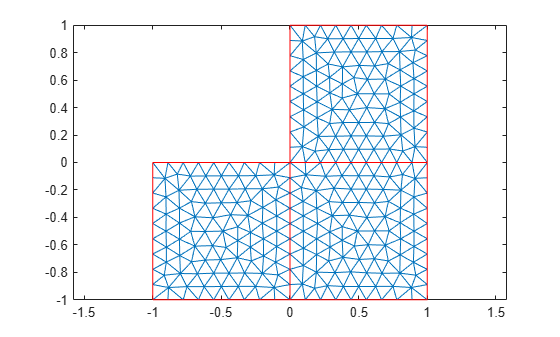Create a mesh that is finer than the default.

Create a PDE model and include the `BracketTwoHoles` geometry.

```model = createpde(1); importGeometry(model,'BracketTwoHoles.stl');```

Generate a default mesh for comparison.

`generateMesh(model)`
```ans = FEMesh with properties: Nodes: [3x10003 double] Elements: [10x5774 double] MaxElementSize: 9.7980 MinElementSize: 4.8990 MeshGradation: 1.5000 GeometricOrder: 'quadratic' ```

View the mesh.

`pdeplot3D(model)`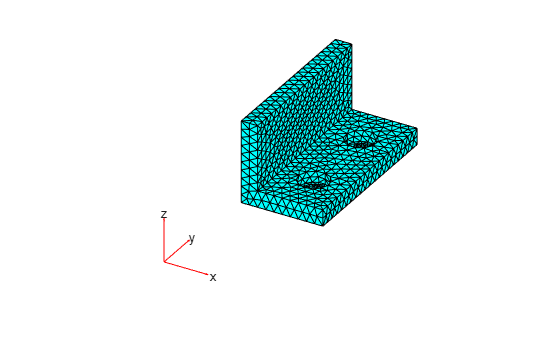Create a mesh with target maximum element size 5 instead of the default 7.3485.

`generateMesh(model,'Hmax',5)`
```ans = FEMesh with properties: Nodes: [3x66982 double] Elements: [10x44093 double] MaxElementSize: 5 MinElementSize: 2.5000 MeshGradation: 1.5000 GeometricOrder: 'quadratic' ```

View the mesh.

`pdeplot3D(model)`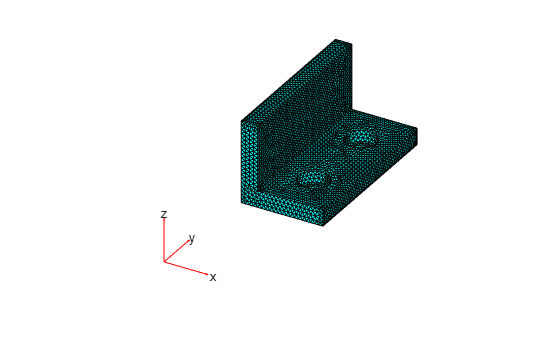Generate a 2-D mesh with finer spots around the specified edges and vertices.

Create a model.

`model = createpde;`

Create and plot a 2-D geometry representing a circle with a diamond-shaped hole in its center.

```g = geometryFromEdges(model,@scatterg); pdegplot(g,'VertexLabels','on','EdgeLabels','on')```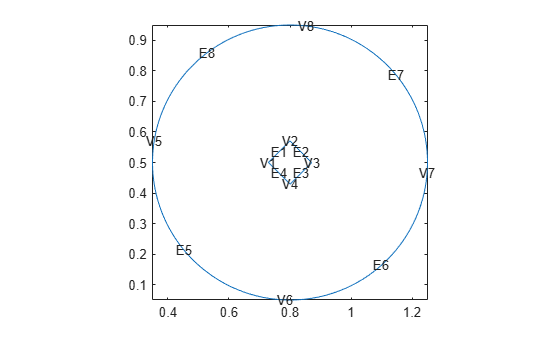Generate a mesh for this geometry using the default mesh parameters.

`m1 = generateMesh(model)`
```m1 = FEMesh with properties: Nodes: [2x1159 double] Elements: [6x547 double] MaxElementSize: 0.0509 MinElementSize: 0.0254 MeshGradation: 1.5000 GeometricOrder: 'quadratic' ```

Plot the resulting mesh.

`pdeplot(m1)`Generate a mesh with the target size on edge 1, which is smaller than the target minimum element size, `MinElementSize`, of the default mesh.

`m2 = generateMesh(model,'Hedge',{1,0.001})`
```m2 = FEMesh with properties: Nodes: [2x2631 double] Elements: [6x1241 double] MaxElementSize: 0.0509 MinElementSize: 0.0254 MeshGradation: 1.5000 GeometricOrder: 'quadratic' ```

Plot the resulting mesh.

`pdeplot(m2)`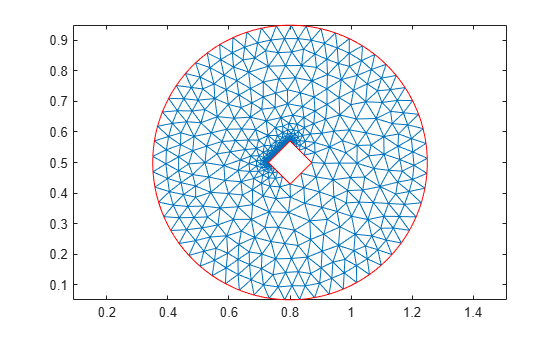Generate a mesh specifying the target sizes for edge 1 and vertices 6 and 7.

`m3 = generateMesh(model,'Hedge',{1,0.001},'Hvertex',{[6 7],0.002})`
```m3 = FEMesh with properties: Nodes: [2x2903 double] Elements: [6x1365 double] MaxElementSize: 0.0509 MinElementSize: 0.0254 MeshGradation: 1.5000 GeometricOrder: 'quadratic' ```

Plot the resulting mesh.

`pdeplot(m3)`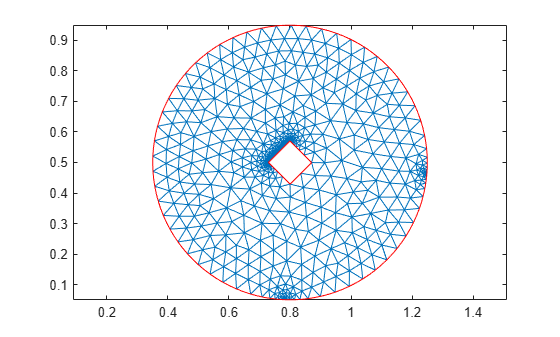## Input Arguments

collapse all

Model object, specified as a `PDEModel` object, `ThermalModel` object, `StructuralModel` object, or `ElectromagneticModel` object.

Example: `model = createpde(3)`

Example: `thermalmodel = createpde('thermal','steadystate')`

Example: `structuralmodel = createpde('structural','static-solid')`

Example: ```emagmodel = createpde('electromagnetic','electrostatic')```

### Name-Value Arguments

Specify optional comma-separated pairs of `Name,Value` arguments. `Name` is the argument name and `Value` is the corresponding value. `Name` must appear inside quotes. You can specify several name and value pair arguments in any order as `Name1,Value1,...,NameN,ValueN`.

Example: `generateMesh(model,'Hmax',0.25);`

Element geometric order, specified as `'``linear'` or `'quadratic'`.

A triangle or tetrahedron representing a linear element has nodes at the corners. A triangle or tetrahedron representing a quadratic element has nodes at its corners and edge centers. The center nodes in quadratic meshes are always added at half-distance between corners. For geometries with curved surfaces and edges, center nodes might not appear on the edge or surface itself.

In general, `'quadratic'` elements produce more accurate solutions. Override the default `'quadratic'` only to solve a 3-D magnetostatic problem, to save memory, or to solve a 2-D problem using a legacy solver. Legacy PDE solvers use linear triangular mesh for 2-D geometries.

Example: `generateMesh(model,'GeometricOrder','linear');`

Data Types: `char` | `string`

Mesh growth rate, specified as a number greater than or equal to 1 and less than or equal to 2.

Example: `generateMesh(model,'Hgrad',1.3);`

Data Types: `double`

Target maximum mesh edge length, specified as a positive number.

`Hmax` is an approximate upper bound on the mesh edge lengths. Occasionally, `generateMesh` can create a mesh with some elements that exceed `Hmax`.

`generateMesh` estimates the default value of `Hmax` from overall dimensions of the geometry.

Small `Hmax` values let you create finer meshes, but mesh generation can take a very long time in this case. You can interrupt mesh generation by using Ctrl+C. Note that `generateMesh` can take additional time to respond to the interrupt.

Example: `generateMesh(model,'Hmax',0.25);`

Data Types: `double`

Target minimum mesh edge length, specified as a nonnegative number.

`Hmin` is an approximate lower bound on the mesh edge lengths. Occasionally, `generateMesh` can create a mesh with some elements that are smaller than `Hmin`.

`generateMesh` estimates the default value of `Hmin` from overall dimensions of the geometry.

Example: `generateMesh(model,'Hmin',0.05);`

Data Types: `double`

Target size on selected faces, specified as a cell array containing an even number of elements. Odd-indexed elements are positive integers or vectors of positive integers specifying face IDs. Even-indexed elements are positive numbers specifying the target size for the corresponding faces.

Example: ```generateMesh(model,'Hmax',0.25,'Hface',{[1 2],0.1,[3 4 5],0.05})```

Data Types: `double`

Target size around selected edges, specified as a cell array containing an even number of elements. Odd-indexed elements are positive integers or vectors of positive integers specifying edge IDs. Even-indexed elements are positive numbers specifying the target sizes for the corresponding edges.

Example: ```generateMesh(model,'Hmax',0.25,'Hedge',{[1 2],0.01,3,0.05})```

Data Types: `double`

Target size around selected vertices, specified as a cell array containing an even number of elements. Odd-indexed elements are positive integers or vectors of positive integers specifying vertex IDs. Even-indexed elements are positive numbers specifying the target sizes for the corresponding vertices.

Example: `generateMesh(model,'Hmax',0.25,'Hvertex',{1,0.02})`

Data Types: `double`

## Output Arguments

collapse all

Mesh description, returned as an FEMesh Properties object. `mesh` is the same as `model.Mesh`.

collapse all

### Element

An element is a basic unit in the finite-element method.

For 2-D problems, an element is a triangle in the `model.Mesh.Element` property. If the triangle represents a linear element, it has nodes only at the triangle corners. If the triangle represents a quadratic element, then it has nodes at the triangle corners and edge centers.

For 3-D problems, an element is a tetrahedron with either four or ten points. A four-point (linear) tetrahedron has nodes only at its corners. A ten-point (quadratic) tetrahedron has nodes at its corners and at the center point of each edge.

For details, see Mesh Data.

## Tips

• `generateMesh` can return slightly different meshes in different releases. For example, the number of elements in the mesh can change. Avoid writing code that relies on explicitly specified node and element IDs or node and element counts.

• `generateMesh` uses the following set of rules when you specify local element sizes with `Hface`, `Hedge`, or `Hvertex`. These rules are valid for both the default and custom values of `Hmin` and `Hmax`.

• If you specify local sizes for regions near each other, `generateMesh` uses the minimum size. For example, if you specify size 1 on an edge and size 0.5 on one of its vertices, the function gradually reduces the element sizes in the proximity of that vertex.

• If you specify local sizes smaller than `Hmin`, `generateMesh` ignores `Hmin` in those localities.

• If you specify local sizes larger than `Hmax`, `generateMesh` ignores the specified local sizes. `Hmax` is not exceeded anywhere in the mesh.